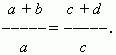# Proportions

Proportion. Crosswise lied terms. Ratios.
Overturned ratios. Derived proportions.

Proportion is an equality of two ratios. From a proportion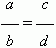follows:
ad = bc (cross products are equal). Inversely, from ad = bc the next proportions follow: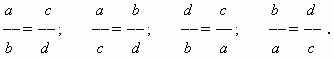All these proportions and some others can be received from the original proportion
a / b = c / d by the next rules:

Crosswise lied terms of any proportion can be changed by places.

Ratios in any proportion can be changed to overturned ones.

Derived proportions .  If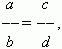then the next so called derived proportions, received from the original, also take place: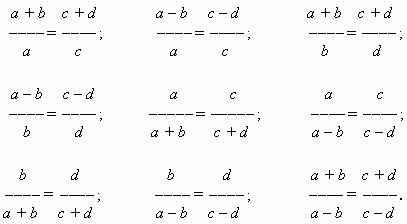All these and a lot of other proportions can be united by the two base formulas: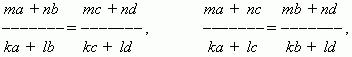where m, n, k, l – any numbers.

E x a m p l e :   If m = n = k = 1, l = 0,  then we receive: